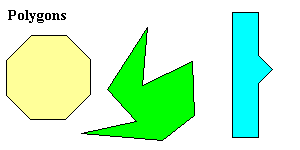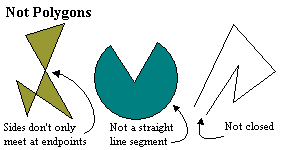# Polygons

A polygon (Greek for many sides ) is a closed figure drawn in the plane using straight line segments which meet only at their endpoints.Polygons are named according to their number of sides.

 Name Number of Sides Triangle $3$ Quadrilateral $4$ Pentagon $5$ Hexagon $6$ Heptagon $7$ Octagon $8$ Nonagon $9$ Decagon $10$ Hendecagon (or Undecagon, or Unidecagon) $11$ Dodecagon $12$ $n$ -gon $n$

A regular polygon is one in which all interior angles are congruent and all sides are congruent; for example, an equilateral triangle, or a square.